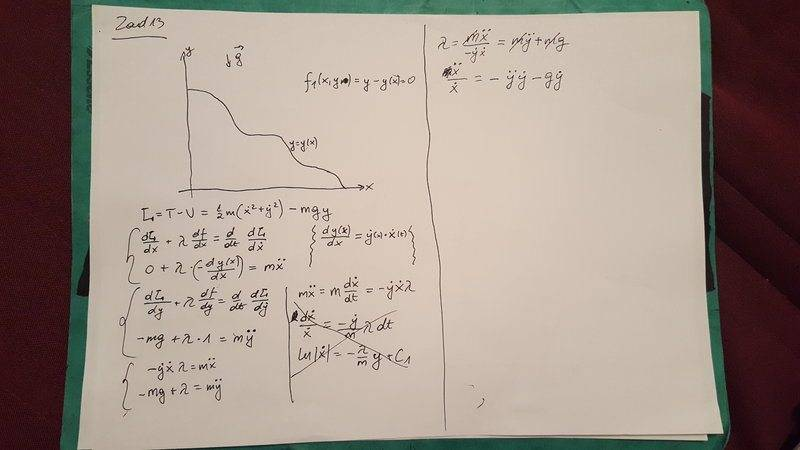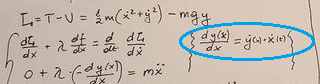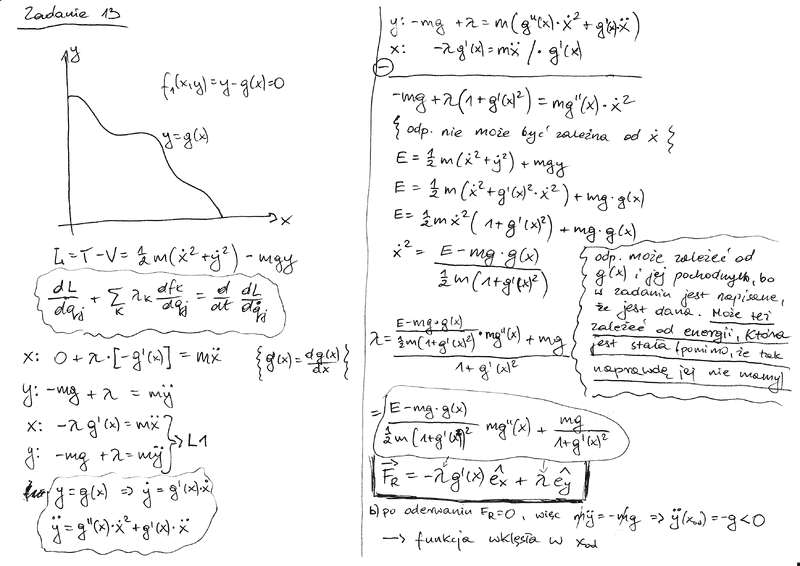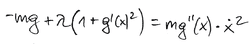# Lagrange Multipliers in Classical Mechanics - exercise 1

mcaay

## Homework Statement

The skier is skiing without friction down the mountain, being all the time in a specified plane. The skier's altitude y(x) is described as a certain defined function of parameter x, which stands for the horizontal distance of the skier from the initial position. The skier is subjected to gravity g = -g ey.

a) Calculate forces of constraint in relation to x.
b) Prove that if the skier can detach from the mountain at a point with horizontal value xod, then y''(xod) < 0, i.e. function y(x) is concave in this point.

Use first kind Lagrange equations (Lagrange multipliers).

## The Attempt at a SolutionTo be honest I am completely lost. This is so abstract with not even a function given that I don't know how to specify the initial constraint equation. Normally there would be y and x in it, so I wrote f(x,y) = y - y(x) = 0, which feels weird ...

I wrote the Lagrange equations based on that weird constraint, but even if that would be good I have no idea how to get Lambda from that :(

I started doing masters in physics after finishing bachelor in mechatronics, and compared to physics students I have huge gaps in knowledge (even though classical mechanics is a 2nd year subject here).

#### Attachments

Homework Helper
Gold Member
Hello.

It is preferable to type in your work rather than post pictures of your work. That way, it is much easier for helpers to quote specific parts of your work. (Posting pictures of diagrams is fine.)

I think you are on the right track, overall. The way you set up the constraint equation as ##f(x, y) = y - y(x) = 0## looks correct.

However, the equation circled below is not correct#### Attachments

•mcaay
mcaay
Hey, sorry for not responding immediately. Thanks for the tips, I think now I got it right, so I'll upload it for people who might lurk in this thread.

Sorry for the Polish comments, I wrote that the answer can not depend on dx/dt, but it can depend on Energy which is constant and on derivatives of g(x).
And in b) that when the skier detaches from the slope - there are no reaction forces, so my'' = -mg#### Attachments

Homework Helper
Gold Member
OK. Part (a) looks good to me. For (b) you showed that ##\ddot y(x_{od}) < 0##. Did you show that ##y''(x_{od}) < 0## ?

•mcaay
mcaay
Oh damn! Thanks for lettng me realize it's not the same :p

Homework Helper
Gold Member
Yes. Or you could let ##\lambda = 0## in#### Attachments

•mcaay
Homework Helper
Dearly Missed
Hey, sorry for not responding immediately. Thanks for the tips, I think now I got it right, so I'll upload it for people who might lurk in this thread.

Sorry for the Polish comments, I wrote that the answer can not depend on dx/dt, but it can depend on Energy which is constant and on derivatives of g(x).
And in b) that when the skier detaches from the slope - there are no reaction forces, so my'' = -mg

View attachment 215647
STOP posting images---it is against PF standards. Do what others have done: take the time and effort to type it out.

•Orodruin